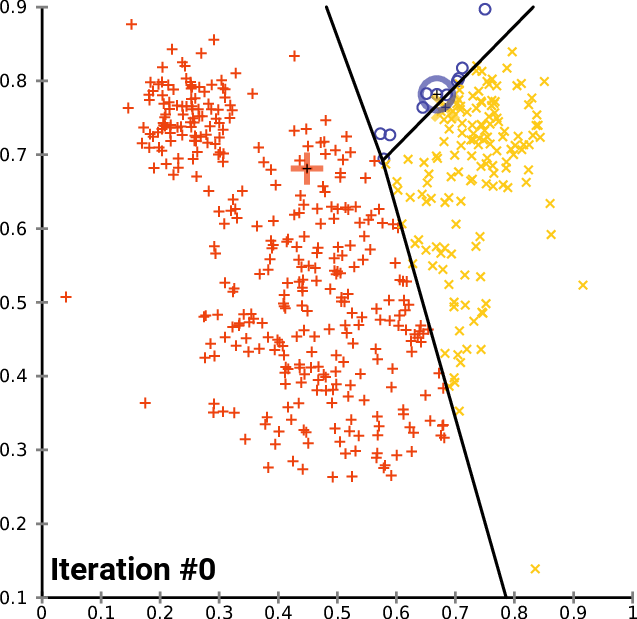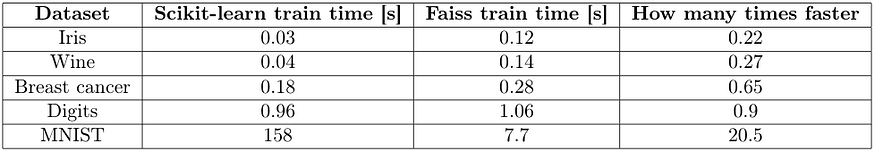## K-Means 8x faster, 27x lower error than Scikit-learn in 25 lines

By Jakub Adamczyk, Computer science student, Python and Machine Learning enthusiast..In my last article on the faiss library, I showed how to make kNN up to 300 times faster than Scikit-learn’s in 20 lines using Facebook’s faiss library. But we can do much more with it, including both faster and more accurate K-Means clustering, in just 25 lines!

K-Means is an iterative algorithm, which clusters the data points into k clusters, each represented with a mean/center point (a centroid). Training starts with some initial guesses and then alternates between two steps: assignment and update.

In the assignment phase, we assign each point to the nearest cluster (using Euclidean distance between point and centroids). In the update step, we recalculate each centroid by calculating a mean point from all points assigned to that cluster in the current step.

The final quality of clustering is calculated as a sum of in-cluster distances, where for each cluster, we calculate a sum of Euclidean distances between points in that cluster and its centroid. This is also called inertia.

For prediction, we perform a 1-nearest neighbor search (kNN with k = 1) between new points and centroids.

### Scikit-learn vs faiss

In both libraries, we have to specify algorithm hyperparameters: number of clusters, number of restarts (each starting with other initial guesses), and maximal number of iterations.

As we can see from the example, the core of the algorithm is searching for the nearest neighbors, specifically the nearest centroids, both for training and prediction. And that’s where faiss is orders of magnitude faster than Scikit-learn! It leverages great C++ implementation, concurrency wherever possible, and even the GPU, if you want.

### Implementing K-Means clustering with faiss

A great feature of faiss is that it has both installation and build instructions and excellent documentation with examples. After the installation, we can write the actual clustering. The code is quite simple because we just mimic the Scikit-learn API.

Important elements:

• faiss has a built-in Kmeans class specifically for this task, but its arguments have different names than in Scikit-learn (see the docs)
• we have to make sure we use np.float32 type, as faiss only works with this type
• kmeans.obj returns a list of errors through the training, so to get only the final one, like in Scikit-learn, we use the [-1] index
• prediction is done with the Index data structure, which is the basic building block of faiss, and is used in all nearest neighbor searches
• in prediction, we perform the kNN search with k = 1, returning indices of nearest centroids from self.cluster_centers_ (index , because index.search() returns distances and indices)

### Time and accuracy comparison

I’ve chosen a few popular datasets available in Scikit-learn for comparison. The train and predict times are compared. For easier reading, I’ve explicitly written how many times faster is the faiss-based clustering than Scikit-learn’s. For error comparison, I’ve just written how many times lower error the faiss-based clustering achieves (because numbers are large and not very informative).

All of these times have been measured with the time.process_time() function that measures process time instead of wall clock time, for more accurate results. Results are averages of 100 runs, except for MNIST, where it took too long for Scikit-learn, and I had to do 5 runs.Train times (image by author).Predict times (image by author).Training errors (image by author).

As we can see, for K-Means clustering for small datasets (first 4 datasets) faiss-based version is slower for training and has a larger error. For prediction, it works universally faster.

For the larger MNIST dataset, faiss is a clear winner. Training 20.5 times faster is huge, especially because it reduces time from almost 3 minutes to less than 8 seconds! A 1.5 times faster prediction is also nice. The true achievement, however, is a spectacular 27.5 times lower error. This means that for a larger, real-world dataset, the faiss-based version is vastly more accurate. And this takes only 25 lines of code!

So based on this: if you have a large (at least a few thousand samples) real-world dataset, the faiss-based version is just plain better. For a small toy dataset, Scikit-learn is a better choice; however, if you have a GPU, the GPU-accelerated faiss version may turn out faster (I haven’t checked it for fair CPU comparison).

### Summary

With 25 lines of code, we can get a huge speed and accuracy boost for K-Means clustering for reasonably sized datasets with the faiss library. If you need, you can get even better with a GPU, multiple GPUs, and more, which is nicely explained in the faiss docs.

Original. Reposted with permission.

Related: